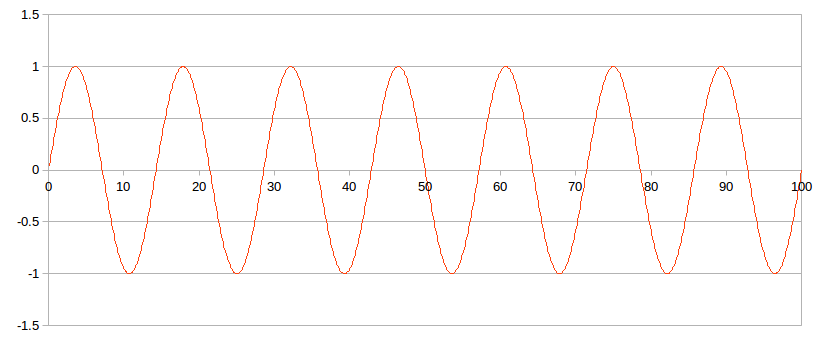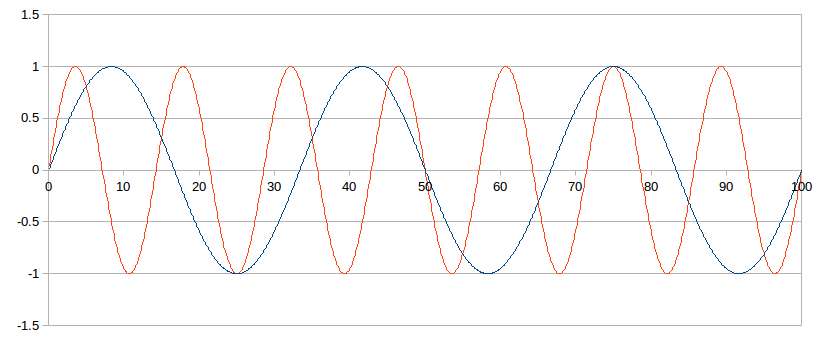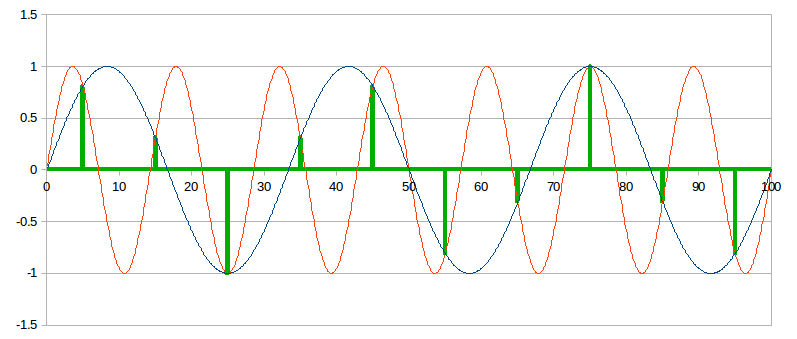# DACs and Digital Filters, Pushing the Limits

I’ve discussed this topic before, here and here. A recent discussion at ASR led me to think about this further, devise some practical examples, and gain a deeper understanding, which I share here.

44-16 is a Tough Nut to Crack

It all started with the digital filters of the WM8741, which my DAC uses (article linked above). We tend to think of CD audio as being “perfect” for all practical purposes. It certainly is higher quality than lossless streaming, and perceptually transparent for most people. Yet at 44.1 kHz, none of the WM8741’s 5 filters was perfect from an engineering perspective. The closest were filters #3 and #5, which it labels “sharp linear phase” and “slow linear phase”, respectively.

Filter #3 has perfectly flat frequency response up to 20,021 Hz (0.454 fs at 44,100 kHz sampling) and no phase distortion. Problem is, it is too weak. At Nyquist (22,050) it is attenuated only 6.43 dB and the stopband (-110 dB) is 24,079 Hz (0.546 fs at 44,100 kHz sampling). The stopband being above Nyquist, it could allow high frequency noise to leak through.

Filter #5 is fully attenuated by Nyquist – the stopband (-110 dB) is 22,050 Hz. And it has no phase distortion. But the passband only goes up to 18,390 Hz, so it begins to attenuate below 20 kHz.

Neither of these filters is perfect, each is a compromise. Why is that? The problem is, the CD standard of 44.1 kHz sampling is so low, it forces a filter transition band that is very narrow (20,000 to 22,050; only 0.14 octaves). Even with modern hardware, it’s hard to implement digital filters that are correct from an engineering perspective and run in real-time, with these constraints. Something’s got to give: frequency response, phase response, or Nyquist attenuation.

Note that at 48 kHz, the WM8741’s filter is perfect. Fully attenuated at Nyquist, with no attenuation or phase shift below 20 kHz. So while 44.1 kHz may not be quite sufficient for implementing perfect real-time filters, it’s almost sufficient. It only takes just a little more “room” to make it perfect. By “room” I mean a wider filter transition band.

So which of these filters, #3 or #5, is better? At first I thought filter #5 was better because I considered full attenuation at Nyquist to be the most important feature of any digital reconstruction filter. Few people (not me) can hear above 18 kHz, so that is a small price to pay for full attenuation. But on further thought, I believe that filter #3 is better. To explain why, I’ll start with aliasing.

Aliasing

Most audiophiles have heard of aliasing and have some idea what it means. Yet surprisingly few have a solid grasp on the math behind what it actually is. I was one of them, so I did a little exploring to rectify that.

The Nyquist-Shannon theorem says if we sample at least twice as fast as the highest frequency we want to capture, our sampling points capture the wave with mathematical perfection. The Whittaker Shannon formula provides a method to perfectly reconstruct the analog wave from the digital sampling points. In both cases, limiting the bandwidth to frequencies below half the sampling rate (the Nyquist limit) is critical.

`Note: the Whittaker-Shannon interpolation formula provides mathematically perfect reconstruction, but it is not the only way to reconstruct the analog wave. It requires summing an infinite series for every sampling point, and even when the series is truncated it is too computationally expensive to be practical for real-time decoding. Two common methods that DACs use are delta-sigma and R2R, which provide similar results. One can think of these as engineering compromises: mathematically imperfect, but requiring fewer computations.`

For any frequency (below Nyquist) we encode digitally into sampling points, an alias is a different frequency (above Nyquist) that passes through the exact same sampling points. We can derive a mathematical relationship between frequencies and their aliases. Intuitively, each frequency and its alias are reflected across Nyquist. Put differently, they are equidistant from Nyquist, or that Nyquist is always the arithmetic average of a frequency and its alias.

At CD sampling at 44,100 Hz, Nyquist is 22,050 Hz, so we can encode any frequency below this. Examples:

• The alias of 18,000 Hz is 22,050 + (22,050 – 18,000) = 26,100 Hz. That is: 18,000 and 26,100 are each 4,050 away from 22,050: one below it, one above it.
• The alias of 1 kHz is 43,100 Hz; each is 21,050 away from Nyquist
• The alias of 100 Hz is 44,000 Hz; each is 21,950 away from Nyquist

A picture’s worth a thousand words. In the following graphs, I use small numbers to keep it all simple, but it all extends to any sampling frequency. The entire X axis is 1 second, and we sample at 10 Hz, so Nyquist is 5 Hz.

Here is a 3 Hz wave.At 10 Hz sampling, the alias of this 3Hz wave is 7 Hz, in red below.Now recall what exactly it means to say that these 2 waves are aliases of each other at 10 Hz sampling: it means either of these waves can perfectly match the same sampling points.

We can see this below:Hmmm… is that not obvious? OK try this:The green shows the points where these waves intersect. Of course, intersecting means they are equal. Observe that these intersection points are perfectly evenly spaced in time. If you sampled either of these waves at these points, you would get the exact same thing. Both waves perfectly fit the sampling points. That is what aliasing means.

`Note: the astute reader may notice that the above 2 waves intersect more often than the points noted in green. For purposes of digital sampling and reconstruction, it is sufficient that they pass through the same sampling points, and it's irrelevant whether they intersect more often than that.`

Now suppose all you have are these sampling points, and you must construct the analog wave. You could construct either one! So the solution is ambiguous: how do you know which is the correct one — meaning the one that was recorded and encoded?

Recall the primary rule of digital recording: you must filter the analog wave to remove all frequencies above Nyquist. The same rule applies when reconstructing the wave from the sampling points. Alias pairs are always symmetrically centered around Nyquist; one above, one below. Thus, filtering to only frequencies below Nyquist eliminates the ambiguity during reconstruction.

A Simple Yet Clever Trick

One conclusion we can draw from the above is that frequencies close to Nyquist have aliases close to Nyquist. Grokking the fullness of this symmetry leads to a simple, yet clever trick when implementing digital reconstruction filters.

As we’ve seen above, the filter’s stopband should be no higher than Nyquist. But squashing the signal from full scale at 20,000 Hz to negative infinity (say -100 dB) at 22,050 Hz will cause passband artifacts, given real-time hardware limitations.

Yet consider what happens if we break the rules and shift the filter stopband a little above Nyquist. Remember how aliases reflect across Nyquist? We want the top of our passband to be 20 kHz, and Nyquist is 22,050. The difference is 2,050 Hz. Add that to Nyquist and we have 24,100 Hz. This is the alias of 20 kHz, when sampled at 44.1 kHz. What if we make this the filter stopband?

Any frequency below 20 kHz will have an alias above 24,100 Hz, so it will be fully attenuated. Conversely, any frequency between Nyquist and the stopband will have an alias above 20 kHz. And we stretched our filter transition twice as wide, making a gentler slope, easier to implement.

Thus, our digital filter will be imperfect from a math or engineering perspective, but perceptually transparent. It may leak some frequencies above Nyquist, which is by definition noise or distortion (call it “junk”). But all this “junk” and its aliases must be all above 20 kHz which is inaudible.

In this case, we shifted the filter stopband just a bit above Nyquist, to widen its transition band. We took advantage of aliasing symmetry, or the fact that frequencies near Nyquist have their aliases near Nyquist.

Of course, TANSTAAFL and this is no exception. This filter may leak some supersonic junk from 20 kHz to 24 kHz. This is inaudible in itself, but when it passes through analog circuits (preamps, power amps, speakers), harmonic and intermodulation distortion will create artifacts in the passband. However, this filter transition band from 20 to 24 kHz is strongly attenuated and most music has little or no energy up there to begin with. So pragmatically speaking, it should not be a problem. Even so, one can see why Wolfson’s engineers provided filter #5 as an alternative – being fully attenuated at Nyquist, it cannot leak any supersonic junk. So the engineers building devices that use the WM8741 can choose which filter makes the best compromise for their needs.

The WM8741 Uses this Trick

Now let’s take another look at the WM8741’s filter #3, at 44.1 kHz sampling. The passband goes up to .454 * fs, which is 20,021 Hz. The stopband is .546 * fs, which is 24,079 Hz. The range between them is the transition band.

Notice anything interesting about these numbers? The transition band is perfectly centered around Nyquist! By sampling frequency ratio, it’s .046 below and .046 above. By frequency, it’s 2,029 below and 2,029 above. Any frequency below 20,021 will alias above 24,049, so aliases of all passband frequencies are fully attenuated. This is the filter we just described above!

BTW, I don’t think this trick is unique to the WM8741. At ASR, reviews of various DACs show their “sharp, linear phase” digital filters down only 6 dB at Nyquist (22,050 Hz), and their stopband around 24 kHz. So it seems like common engineering practice, creative rule-breaking to stretch the limits and provide the best implementation possible given the constraints of 44.1 kHz sampling. Now I know why, and so do you!

If audio standardized on a higher sampling frequency (even only slightly higher like 48 kHz which is already used for DVD), or as DAC chips gain more processing power, these engineering compromises would become unnecessary.